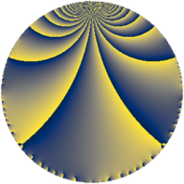# Properties

 Label 4620.2.a.nLevel $4620$ Weight $2$ Character orbit 4620.a Self dual yes Analytic conductor $36.891$ Analytic rank $1$ Dimension $1$ CM no Inner twists $1$

# Related objects

Show commands: Magma / PariGP / SageMath

## Newspace parameters

comment: Compute space of new eigenforms

[N,k,chi] = [4620,2,Mod(1,4620)]

mf = mfinit([N,k,chi],0)

lf = mfeigenbasis(mf)

from sage.modular.dirichlet import DirichletCharacter

H = DirichletGroup(4620, base_ring=CyclotomicField(2))

chi = DirichletCharacter(H, H._module([0, 0, 0, 0, 0]))

N = Newforms(chi, 2, names="a")

//Please install CHIMP (https://github.com/edgarcosta/CHIMP) if you want to run this code

chi := DirichletCharacter("4620.1");

S:= CuspForms(chi, 2);

N := Newforms(S);

 Level: $$N$$ $$=$$ $$4620 = 2^{2} \cdot 3 \cdot 5 \cdot 7 \cdot 11$$ Weight: $$k$$ $$=$$ $$2$$ Character orbit: $$[\chi]$$ $$=$$ 4620.a (trivial)

## Newform invariants

comment: select newform

sage: f = N # Warning: the index may be different

gp: f = lf \\ Warning: the index may be different

 Self dual: yes Analytic conductor: $$36.8908857338$$ Analytic rank: $$1$$ Dimension: $$1$$ Coefficient field: $$\mathbb{Q}$$ Coefficient ring: $$\mathbb{Z}$$ Coefficient ring index: $$1$$ Twist minimal: yes Fricke sign: $$1$$ Sato-Tate group: $\mathrm{SU}(2)$

## $q$-expansion

comment: q-expansion

sage: f.q_expansion() # note that sage often uses an isomorphic number field

gp: mfcoefs(f, 20)

 $$f(q)$$ $$=$$ $$q + q^{3} + q^{5} + q^{7} + q^{9}+O(q^{10})$$ q + q^3 + q^5 + q^7 + q^9 $$q + q^{3} + q^{5} + q^{7} + q^{9} - q^{11} - 4 q^{13} + q^{15} + 3 q^{17} - 7 q^{19} + q^{21} - 9 q^{23} + q^{25} + q^{27} - 3 q^{29} + 2 q^{31} - q^{33} + q^{35} - 4 q^{37} - 4 q^{39} - 6 q^{41} - q^{43} + q^{45} - 6 q^{47} + q^{49} + 3 q^{51} + 3 q^{53} - q^{55} - 7 q^{57} - 9 q^{59} - q^{61} + q^{63} - 4 q^{65} - 10 q^{67} - 9 q^{69} + 6 q^{71} + 8 q^{73} + q^{75} - q^{77} - 4 q^{79} + q^{81} + 3 q^{83} + 3 q^{85} - 3 q^{87} - 15 q^{89} - 4 q^{91} + 2 q^{93} - 7 q^{95} - q^{97} - q^{99}+O(q^{100})$$ q + q^3 + q^5 + q^7 + q^9 - q^11 - 4 * q^13 + q^15 + 3 * q^17 - 7 * q^19 + q^21 - 9 * q^23 + q^25 + q^27 - 3 * q^29 + 2 * q^31 - q^33 + q^35 - 4 * q^37 - 4 * q^39 - 6 * q^41 - q^43 + q^45 - 6 * q^47 + q^49 + 3 * q^51 + 3 * q^53 - q^55 - 7 * q^57 - 9 * q^59 - q^61 + q^63 - 4 * q^65 - 10 * q^67 - 9 * q^69 + 6 * q^71 + 8 * q^73 + q^75 - q^77 - 4 * q^79 + q^81 + 3 * q^83 + 3 * q^85 - 3 * q^87 - 15 * q^89 - 4 * q^91 + 2 * q^93 - 7 * q^95 - q^97 - q^99

## Embeddings

For each embedding $$\iota_m$$ of the coefficient field, the values $$\iota_m(a_n)$$ are shown below.

For more information on an embedded modular form you can click on its label.

comment: embeddings in the coefficient field

gp: mfembed(f)

Label $$\iota_m(\nu)$$ $$a_{2}$$ $$a_{3}$$ $$a_{4}$$ $$a_{5}$$ $$a_{6}$$ $$a_{7}$$ $$a_{8}$$ $$a_{9}$$ $$a_{10}$$
1.1
 0
0 1.00000 0 1.00000 0 1.00000 0 1.00000 0
 $$n$$: e.g. 2-40 or 990-1000 Significant digits: Format: Complex embeddings Normalized embeddings Satake parameters Satake angles

## Atkin-Lehner signs

$$p$$ Sign
$$2$$ $$-1$$
$$3$$ $$-1$$
$$5$$ $$-1$$
$$7$$ $$-1$$
$$11$$ $$1$$

## Inner twists

This newform does not admit any (nontrivial) inner twists.

## Twists

By twisting character orbit
Char Parity Ord Mult Type Twist Min Dim
1.a even 1 1 trivial 4620.2.a.n 1

By twisted newform orbit
Twist Min Dim Char Parity Ord Mult Type
4620.2.a.n 1 1.a even 1 1 trivial

## Hecke kernels

This newform subspace can be constructed as the intersection of the kernels of the following linear operators acting on $$S_{2}^{\mathrm{new}}(\Gamma_0(4620))$$:

 $$T_{13} + 4$$ T13 + 4 $$T_{17} - 3$$ T17 - 3 $$T_{19} + 7$$ T19 + 7

## Hecke characteristic polynomials

$p$ $F_p(T)$
$2$ $$T$$
$3$ $$T - 1$$
$5$ $$T - 1$$
$7$ $$T - 1$$
$11$ $$T + 1$$
$13$ $$T + 4$$
$17$ $$T - 3$$
$19$ $$T + 7$$
$23$ $$T + 9$$
$29$ $$T + 3$$
$31$ $$T - 2$$
$37$ $$T + 4$$
$41$ $$T + 6$$
$43$ $$T + 1$$
$47$ $$T + 6$$
$53$ $$T - 3$$
$59$ $$T + 9$$
$61$ $$T + 1$$
$67$ $$T + 10$$
$71$ $$T - 6$$
$73$ $$T - 8$$
$79$ $$T + 4$$
$83$ $$T - 3$$
$89$ $$T + 15$$
$97$ $$T + 1$$